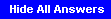### 6.02 Quiz #1 Review Problems

Problem .

Determine the output y[n] for a systems with the input x[n] and unit-sample response h[n] shown below. Assume h[n]=0 and x[n]=0 for any times n not shown.```y[n] = Σx[k]h[n-k] = xh[n] + xh[n-1] + xh[n-2]
= δ[n+1] + 4δ[n] + 8δ[n-1] + 8δ[n-2] + 3δ[n-3]```

Problem . A discrete-time linear system produces output v when the input is the unit step u. What is the output h when the input is the unit-sample δ? Assume v[n]=0 for any times n not shown below.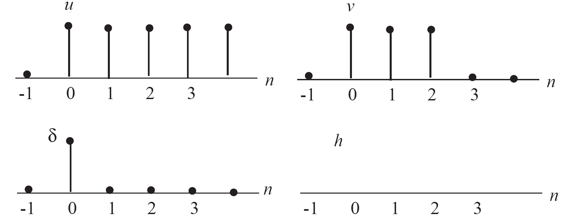The system is causal, so h[n]=0 for n<0.

```v[n] = Σu[k]h[n-k] = h[n] + h[n-1] + h[n-2] + ...
h[n] = v[n] - h[n-1] - h[n-2] - ...

h = v - h[-1] - ... = v = 1
h = v - h - h[-1] - ... = v - h = 1 - 1 = 0
h = v - h - h = 1 - 1 - 0 = 0
h = v - h - h - h = 0 - 0 - 0 - 1 = -1
h = v - h - h - h - h = 0 - (-1) - 0 - 0 - 1 = 0
h[n] = 0, n ≥ 4```Problem .

A signal is transmitted at 20 samples/bit and 8 bits/symbol.

1. How many voltage samples are sent for each symbol? 160 samples/symbol.

2. To convert the samples to bits, where should the receiver sample? The first half of the samples? Or the second half of the samples? Why? Signal transitions over most channels take at least several sample times to complete; for slow channels transitions may take many sample times. So it is better to sample at the latter half of the samples, but not too close to the end since slow transitions make us uncertain about exactly where the sample sequence for a particular bit started, which means we are also uncertain as to where the sequence ends.

3. According to your response in part b), pick a sample number (out of the 20 samples/bit) at which to sample. A list of samples, representing exactly one 8-bit character, has been transmitted. Write a python procedure receive that converts the list of samples into an 8-bit list representation of the 8 bits. Try to do this without using a for loop. Hint: List comprehension.
```def receive(samples, samples_per_bit, bits_per_symbol):
index = samples_per_bit*3/4
return samples[index::samples_per_bit]```

Problem .

The following figure show plots of several received waveforms. The transmitter is sending sequences of binary symbols (i.e., either 0 or 1) at some fixed symbol rate, using 0V to represent 0 and 1V to represent 1.The horizontal grid spacing is 1 microsecond (1e-6 sec).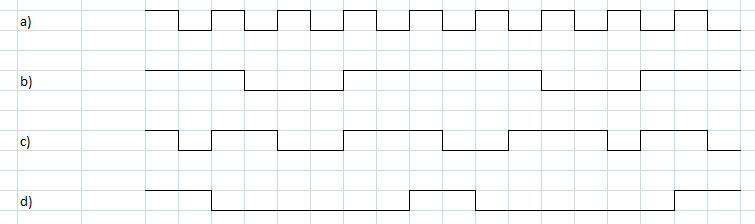Answer the following questions for each plot:

1. Find the slowest symbol rate that is consistent with the transitions in the waveform.

2. Using your answer in question 1, what is the decoded bit string?
a) 1e6 symbols/sec, 101010101010101010
b) 333,333 symbols/sec, 101101
c) 1e6 symbols/sec, 101100111001110110
d) 5e5 symbols/sec, 100010001

Problem .

Ben Bitdiddle is doing a 6.02 lab on understanding the effect of noise on data receptions, and is confused about the following questions. Please help him by answering them.

In these questions, assume that:

1. The sender sends 0 Volts for a "0" bit and 1 Volt for a "1" bit
2. P_ij = Probability that a bit transmitted as "i" was received as a "j" bit (for all four combinations of i and j, 00, 01, 10, 11)
3. alpha = Probability that the sender sent bit 0
4. beta = Probability that the sender sent bit 1
5. and, obviously, alpha + beta = 1

The channel has non-zero random noise, but unless stated otherwise, assume that the noise has 0 mean and that it is a Gaussian with finite variance. The noise affects the received samples in an additive manner, as in the labs you've done.

1. Which of these properties does the bit error rate of this channel depend on?
1. The voltage levels used by the transmitter to send "0" and "1"
2. The variance of the noise distribution
3. The voltage threshold used to determine if a sample is a "0" or a "1"
4. The number of samples per bit used by the sender and receiver
In general the bit error rate is a function of both noise and inter-symbol interference.

The noise is a function of Φ[(vth - (signal_level + noise_mean))/noise_sigma] multiplied as appropriate by alpha or beta. So the bit error rate clearly depends on the signal level, the mean and variance of the noise and the digitization threshold.

The number of samples per bit doesn't enter directly into the bit error calculation, but more samples per bit gives each transition more time to reach its final value, reducing inter-symbol interference. This means that the eye will be more open. In the presence of noise, a wider eye means a lower bit error rate.

2. Suppose Ben picks a voltage threshold that minimizes the bit error rate. For each choice below, determine whether it's true or false.
1. P_01 + P_10 is minimized for all alpha and beta
2. alpha * P_01 + beta * P_10 is minimized
3. P_01 = P_10 for all alpha and beta
4. if alpha > beta then P_10 > P_01
5. The voltage threshold that minimizes BER depends on the noise variance if alpha = beta
(b) is the definition of bit error rate, so that's clearly minimized. Thus (a) is only minimized if alpha = beta.

The magnitude of the BER is, of course, a function of the noise variance, but for a given noise variance, if alpha = beta, the minimum BER is achieved by setting the digitization threshold at 0.5. So (e) is false.

As we saw in PSet #3, when alpha ≠ beta, the noise is minimized when the digitization threshold moves away from the more probable signal. Suppose alpha > beta. The digitization threshold would increase so P_01 would get smaller and P_10 larger. So (c) is not true and (d) is true.

3. Suppose alpha = beta. If the noise variance doubles, what happens to the bit error rate? When alpha = beta = 0.5, the minimum BER is achieved when the digitization threshold is half-way between the signaling levels, i.e., 0.5V. Using Φ(x), the cumulative distribution function for the unit normal PDF, we can write the following formula for the BER:

```BER = 0.5*(1 - Φ[.5/σ]) + 0.5*Φ[-.5/σ]
= Φ[-.5/σ]```

Doubling the noise variance is the same as multiplying σ by sqrt(2), so the resulting BER would be

BERnew = Φ[-.5/(sqrt(2)*σ)]

The change in the bit error rate is given by BERnew - BER.

Problem .

If a transmitter can generate signals between 0V and 5V, how many digital signaling levels can we have if the noise limit is ±.2V and the receiver requires a .1V forbidden zone for each thresholding operation that it needs to implement? Our digital signaling convention requires a ±.2V region around each signaling level and the regions must be separated by a .1V forbidden zone. That means we must have .2+.1+.2 = .5V between signaling levels. With a 5V signaling range, we can get (5/.5)+1=11 digital signaling levels.

Problem .

In this problem, we will understand the impact of imperfect clocks and clock drift on clock recovery in communication systems. A transmitter and receiver are communicating using a perfect communication channel (that is, ignore the effects of ISI and noise for now). The transmitter transmits every bit using symbols of duration 10 samples each. The receiver also tries to sample the channel at the same frequency as the transmitter to obtain 10 samples for each symbol, and then digitizes the 10th sample of the symbol to recover the transmitted bit. However, the non-ideal clocks at the transmitter and receiver "drift away" from each other, as a result of which the receiver ends up sampling the channel 10 times in only 9 sample periods of the transmitter. To cope with this clock drift, the receiver resets its sample counter on transitions from 0 to 1 or vice versa. What is the maximum number of contiguous 0s or 1s that the data can have (i.e., the maximum number of bits without transitions in between) before one starts to see bit errors due to the clock drift? One should have no more than 9 consecutive bits without a transition in order not to see bit errors. Bit #10 at receiver = Sample #100 at receiver = Sample #90 at sender = last sample of bit #9 at transmitter => bit error.

Problem .

Consider the problem of synchronization to identify the start of a message between a transmitter and receiver. Suppose the designers of the communication system decide to use the sequence of bits '01111110' to signal the start of a message. What property should the designers enforce on the data to ensure correct synchronization? Care should be taken to ensure that the synchronization pattern does not appear in the data. For example, one can get around this problem encoding the data in such a way that the synchronization pattern does not appear in the data stream (e.g., 8b/10b).

Problem .

The output of a particular communication channel is given by

y[n] = αx[n] + βx[n-1] where α > β

1. Is the channel linear? Is it time invariant? To be linear the channel must meet two criteria:

• if we scale the inputs x[n] by some factor k, the outputs y[n] should scale by the same factor.

• if we get y1[n] with inputs x1[n] and y2[n] with inputs x2[n], then we should get y1[n] + y2[n] if the input is x1[n] + x2[n].

It's easy to verify both properities given the channel response above, so the channel is linear.

To be time invariant the channel must have the property that if we shift the input by some number of samples s, the output also shifts by s samples. Again that property is easily verified given the channel response above, so the channel is time invariant.

2. What is the channel's unit-sample response h? The unit-sample input is x=1 and x[n]=0 for n≠0.

Using the channel response given above, the channel's unit-sample reponse can be computed as

```h=α,
h=β,
h[n]=0 for all other values of n```

3. If the input is the following sequence of samples starting at time 0:

`x[n] = [1, 0, 0, 1, 1, 0, 1, 1], followed by all 1's.`

then what is the channel's output assuming α=.7 and β=.3? Convolving x[n] with h[n] we get

`y[n] = [.7, .3, 0, .7, 1, .3, .7, 1], followed by all 1's.`

4. Again let α=.7 and β=.3. Derive a deconvolver for this channel and compute the input sequence that produced the following output:

`y[n] = [.7, 1, 1, .3, .7, 1, .3, 0], followed by all 0's.`
`w[n] = (1/h)(y[n] - hw[n-1]) = y[n]/.7 - (.3/.7)w[n-1]`

so

`w[n] = [1, 1, 1, 0, 1, 1, 0, 0], followed by all 0's`

Problem .

Suppose four different wires {I,II,III,IIII} have four different unit sample responses:

h1 = .25, .25, .25, .25, 0, ...h2 = 0, .25, .5, .25, 0, ...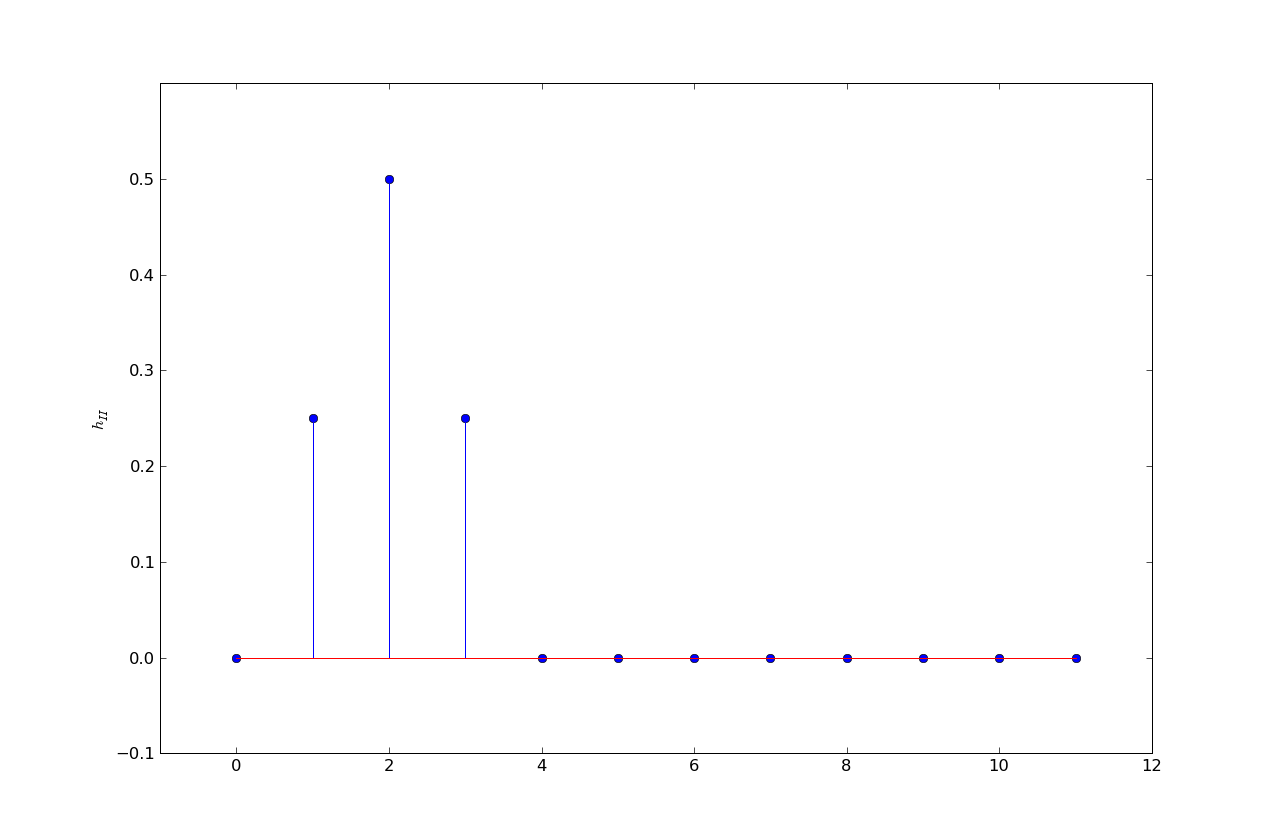h3 = .11, .22, .33, .22, .11, 0, ...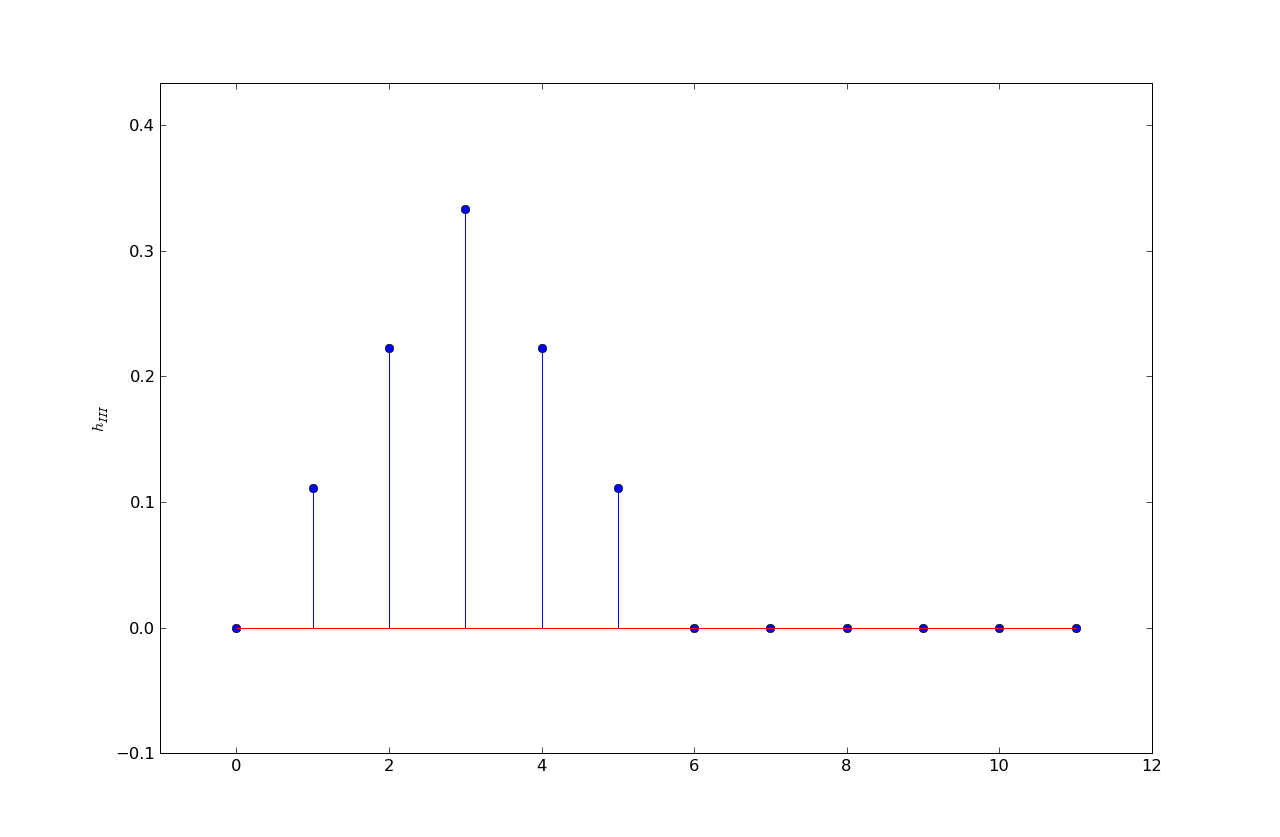h4 = .04, .08, .12, .16, .20, .12, .12, .12, .04, 0, ...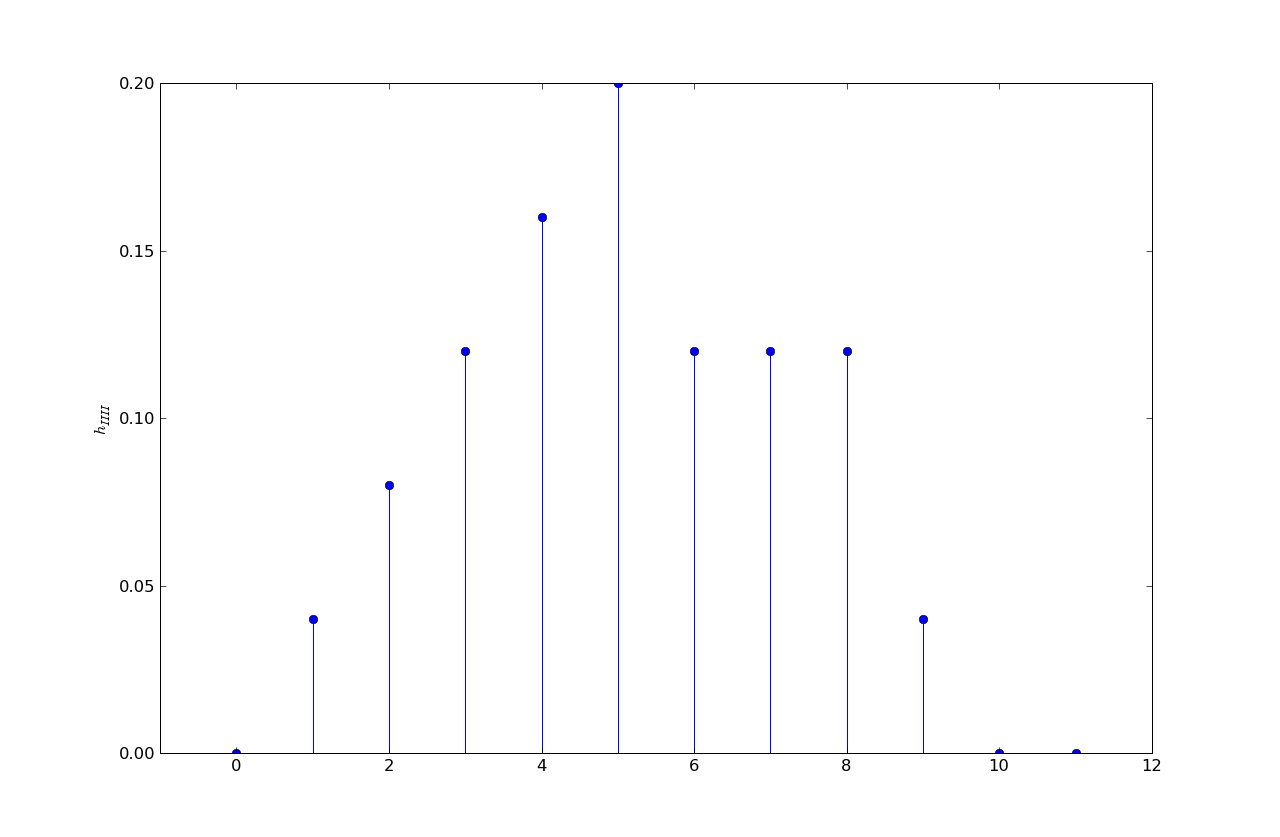Each of the following eye diagrams is associated with transmitting bits using one of the four wires, where five samples were used per bit. That is, a one bit is five one-volt samples and a zero bit is five zero-volt samples. Please determine which wire was used in each case.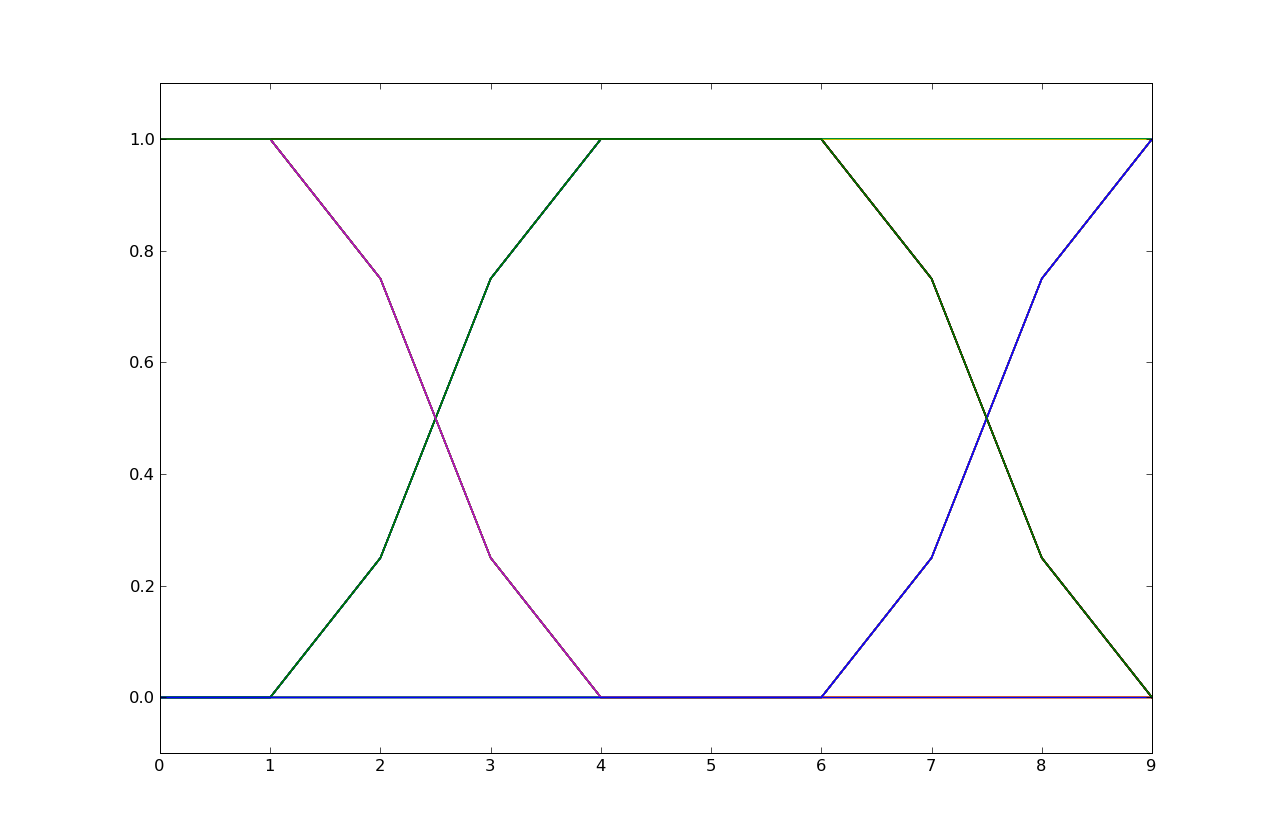The eye diagram is from h2. Note that the signal transitions take three samples to complete and that the transitions occur in 3 steps with a larger slope in the middle step, an indicator of a response with 3 taps with a larger middle tap.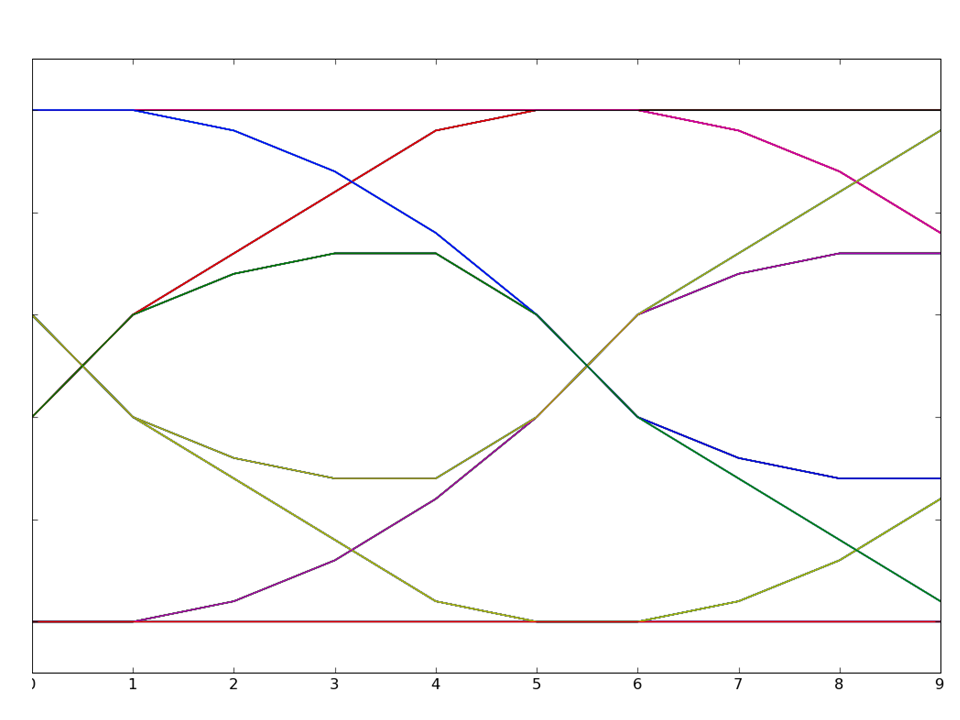The eye diagram is from h4. Note that the signal transitions take more than five samples to complete and hence result in considerable inter-symbol interference. Response h4 is the only response that's non-zero for more than 5 taps.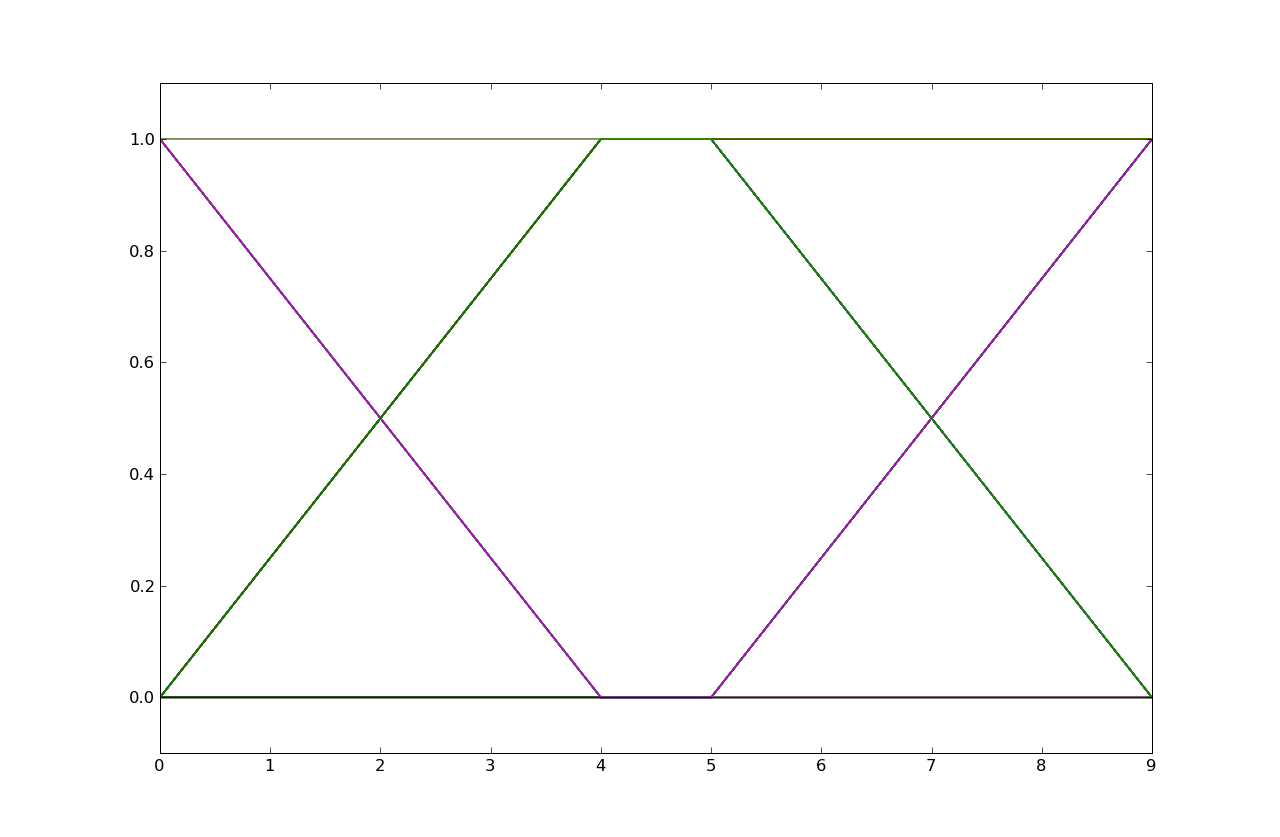The eye diagram is from h1. Note that the signal transitions take four samples to complete and that the transitions have constant slope, an indicator of a response with 4 equal taps.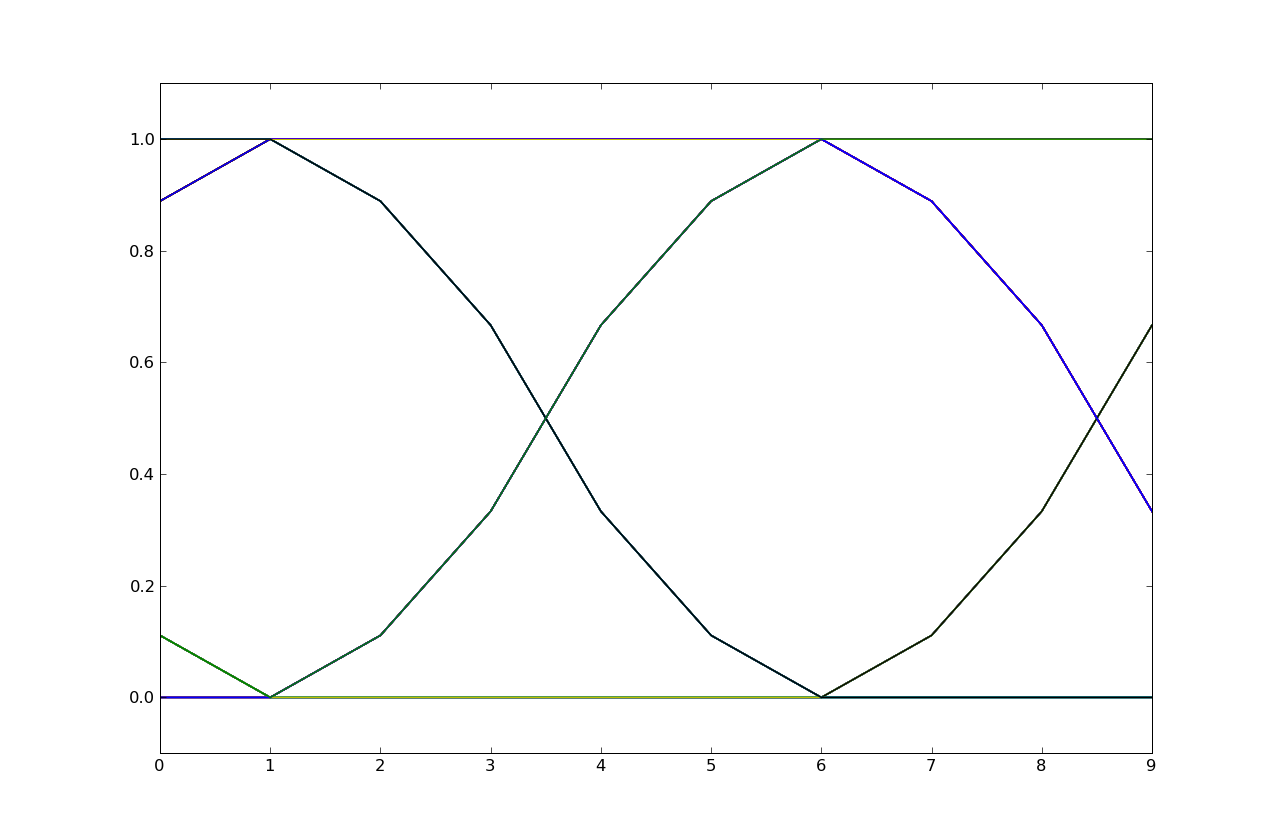The eye diagram is from h3. Note that the signal transitions take five samples to complete and that the transitions occur in 5 steps with larger slopes in the middle of the transition, an indicator of a response with 5 taps with larger middle taps.

Problem .

Consider the second of the four eye diagrams in Problem 10. Determine the eight unique voltage values for sample number 8. If we convolve h4 with a unit step we get the unit-step response:

```n           0    1     2     3     4     5     6     7     8     9
hstep[n] = 0.0, 0.04, 0.12, 0.24, 0.40, 0.60, 0.72, 0.84, 0.96, 1.00, ...```

Now if we consider all possible values of the current bit and the previous two bits (listed earliest-to-latest in the table below) we can use superposition of hstep to compute the possible values at sample time 8, which is the same as asking for y. Note that you have to think about how the sample numbers in the eye diagram align with the sample numbers of the bits -- the eye diagram is not necessary aligned with bit boundaries (e.g., it isn't in this case).

bitsdecomposed into unit stepscomputation for y
1 1 1u[n]y = hstep = 1
1 1 0u[n] - u[n-10]y = hstep - hstep = 1 - .12 = .88
1 0 1u[n] - u[n-5] + u[n-10]y = 1 - .84 + .12 = .28
1 0 0u[n] - u[n-5]y = 1 - .84 = .16
0 1 1u[n-5]y = .84
0 1 0u[n-5] - u[n-10]y = .84 - .12 = .72
0 0 1u[n-10]y = .12
0 0 0 y = 0

So the eight unique values for y are 0, .12, .16, .28, .72, .84, .88 and 1.

Problem .

Messages are transmitted along a noisy channel using the following protocol: a "0" bit is transmitted as -0.5 Volt and a "1" bit as 0.5 Volt. The PDF of the total noise added by the channel, H, is shown below.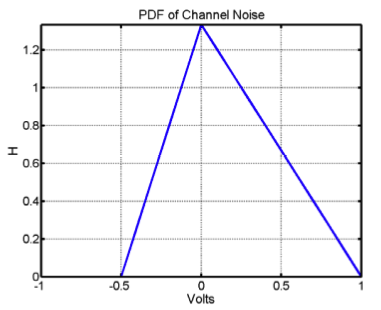1. Compute H(0), the maximum value of H. The area under the PDF is 1, so (0.5)*H(0)*(1+0.5) = 1 from which we get H(0) = 4/3.

2. It is known that a "0" bits 3 times as likely to be transmitted as a "1" bit. The PDF of the message signal, M, is shown below. Fill in the values P and Q.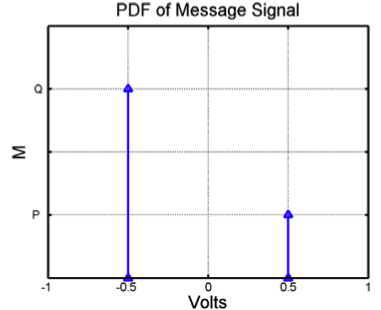We know that P=3Q and that P+Q=1, so P=0.75 and Q=.25.

3. If the digitization threshold voltage is 0V, what is the bit error rate? The plot below shown the PDF of the received voltage in magenta. For a threshold voltage of 0, there is only one error possible: a transmitted "0" received as a "1". This error is equal to the area of the triangle formed by the dotted black line and the blue line = 0.5*0.5*0.5 = 0.125.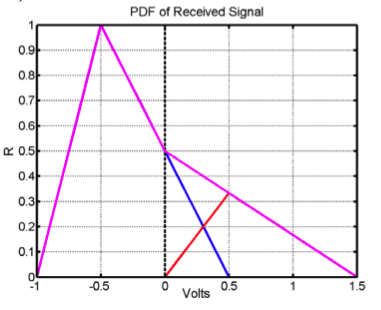4. What digitization threshold voltage would minimize the bit error rate? We'll minimize the bit error rate if the threshold voltage is chosen at the voltage where the red and blue lines intersect. By looking at the plot from the previous answer, let the ideal threshold be x and the value of the PDF at the intersection point be y. Then y/x=2/3 and y/(0.5-x)=1, so x = 0.3V.